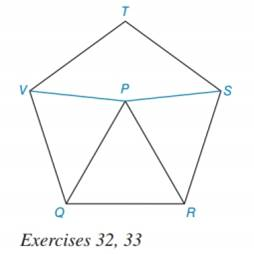Chapter 7.3, Problem 33EElementary Geometry For College St...

7th Edition
Alexander + 2 others
ISBN: 9781337614085

Solutions

Chapter
SectionElementary Geometry For College St...

7th Edition
Alexander + 2 others
ISBN: 9781337614085
Textbook Problem

Given: Regular pentagon RSTVQ with equilateral Δ PQRFind: m ∠ V P STo determine

To find:

The measure of VPS.

Explanation

Given,

The regular pentagon QRSTV

and mPQR is equilateral.

Calculation:

It is given that ΔPQR is an equilateral triangle.

Therefore, mPQR=60

Interior angle of regular polygon is 108°.

mVQR=mVTS=108mVQP=mVQRmPQR=10860=48

Triangle QVP is isosceles as QP=QV.

Let QVP=QPV=x

In triangle QVP

PQV+2x=180

Still sussing out bartleby?

Check out a sample textbook solution.

See a sample solution

The Solution to Your Study Problems

Bartleby provides explanations to thousands of textbook problems written by our experts, many with advanced degrees!

Get Started

Use the Change of Base Formula to evaluate log12 27.

Precalculus: Mathematics for Calculus (Standalone Book)

Subtract: 8yd1ft3in.2yd2ft6in._

Elementary Technical Mathematics

In problems 15-22, simplify by combining like terms. 19.

Mathematical Applications for the Management, Life, and Social Sciences

In Exercises 4562, find the values of x that satisfy the inequality (inequalities). 61. x2x12

Applied Calculus for the Managerial, Life, and Social Sciences: A Brief Approach